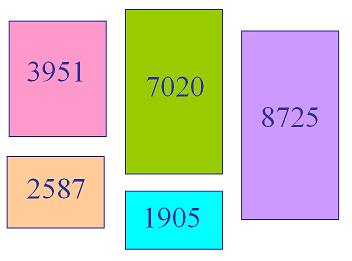#### You may also like### N000ughty Thoughts

How many noughts are at the end of these giant numbers?### DOTS Division

Take any pair of two digit numbers x=ab and y=cd where, without loss of generality, ab > cd . Form two 4 digit numbers r=abcd and s=cdab and calculate: {r^2 - s^2} /{x^2 - y^2}.### Mod 3

Prove that if a^2+b^2 is a multiple of 3 then both a and b are multiples of 3.

# Expenses

##### Age 14 to 16Challenge Level

Expenses printable sheetWhat is the largest number which, when divided into each of $1905$, $2587$, $3951$, $7020$ and $8725$, leaves the same remainder each time?